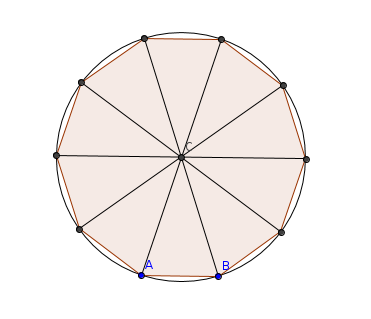# Simple Geometry Problem

Geometry Level 2

If the decagon is inscribed in a unit circle, what is the length of $\overline{AB}$ in the diagram below?×